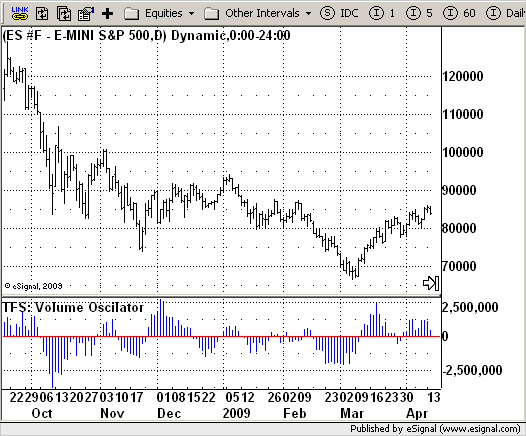# TFS: Volume Oscillator

ICE Data Services -

 TSF_VolOscAvg.efs EFSLibrary - Discussion Board

File Name: TSF_VolOscAvg.efs

Description:
TFS: Volume Oscillator

Formula Parameters:
AvgLen : 7

Notes:
This is the second part of TFS trading strategy. The concept of this
indicator is similar to that of On-Balance Volume indicator (OBV). It
is calculated according to these rules:
If Close > Open, Volume is positive
If Close < Open, Volume is negative
If Close = Open, Volume is neutral
Then you take the 7-day MA of the results.`/*********************************Provided By: eSignal (Copyright c eSignal), a division of Interactive Data Corporation. 2009. All rights reserved. This sample eSignal Formula Script (EFS) is for educational purposes only and may be modified and saved under a new file name. eSignal is not responsible for the functionality once modified. eSignal reserves the right to modify and overwrite this EFS file with each new release.Description: TFS: Volume Oscillator Version: 1.0 05/28/2009 Formula Parameters: Default: AvgLen 7 Notes: This is the second part of TFS trading strategy. The concept of this indicator is similar to that of On-Balance Volume indicator (OBV). It is calculated according to these rules: If Close > Open, Volume is positive If Close < Open, Volume is negative If Close = Open, Volume is neutral Then you take the 7-day MA of the results. **********************************/var fpArray = new Array();var bInit = false;function preMain() { setStudyTitle("TFS: Volume Oscilator"); setCursorLabelName("VolOsc",0); setPlotType(PLOTTYPE_HISTOGRAM, 0); setDefaultBarFgColor(Color.blue, 0); addBand(0, PS_SOLID, 1, Color.red); var x = 0; fpArray[x] = new FunctionParameter("AvgLen", FunctionParameter.NUMBER); with(fpArray[x++]) { setLowerLimit(1); setDefault(7); } }var xTFS_VolumeOsc = null;function main(AvgLen) {var nBarState = getBarState();var nTFS_VolumeOsc = 0; if (nBarState == BARSTATE_ALLBARS) { if (AvgLen == null) AvgLen = 7; } if (bInit == false) { xTFS_VolumeOsc = efsInternal("Calc_TFS_VolumeOsc", AvgLen); bInit = true; } nTFS_VolumeOsc = xTFS_VolumeOsc.getValue(0); if (nTFS_VolumeOsc == null) return; return nTFS_VolumeOsc;}var bSecondInit = false;var xClose = null;var xOpen = null;var xVolume = null;function Calc_TFS_VolumeOsc(AvgLen) {var nRes = 0;var i = 0;var nVolAccum = 0; if (bSecondInit == false) { xClose = close(); xOpen = open(); xVolume = volume(); bSecondInit = true; } if (xClose.getValue(-AvgLen) == null) return; for (i = 0; i < AvgLen; i++) { if (xClose.getValue(-i) > xOpen.getValue(-i)) nVolAccum += xVolume.getValue(-i); if (xClose.getValue(-i) < xOpen.getValue(-i)) nVolAccum -= xVolume.getValue(-i); } nRes = nVolAccum / AvgLen; return nRes;}`